# Representable functor

(diff) ← Older revision | Latest revision (diff) | Newer revision → (diff)

A covariant (or contravariant) functorfrom some category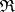into the category of sets(cf. Sets, category of) that is isomorphic to one of the functors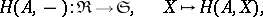or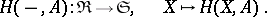A functoris representable if and only if there is an object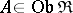and an element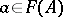such that for every element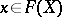,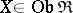, there is a unique morphism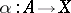for which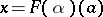. The objectis called a representing object for; it is unique up to isomorphism.

In the category of sets the identity functor is representable: a representing object is a singleton. The functor of taking a Cartesian power is also representable: a representing object is a set whose cardinality equals the given power. In an arbitrary category a product of representable functors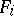with representing objects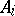,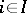, is representable if and only if the coproduct of theexists in the category. Every covariant representable functor commutes with limits, i.e. is continuous (cf. Continuous functor).

A representable functor is an analogue of the concept of a "free universal algebra with one generator" . For any functor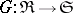and a representable functorthe set of natural transformations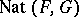is isomorphic to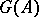, whereis a representing object for. This shows that representable functors are free objects in the category of functors.

In the case of additive categories one considers additive functors with values in the category of Abelian groups instead of functors with values in. Therefore, in this case one understands a representable functor to be an additive functor isomorphic to one of the form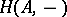or.

The concept of a representable functor arose first in algebraic geometry (cf. ). The most important examples of representable functors in this branch are Picard functors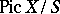and Hilbert functors, which are representable in the category of algebraic spaces (cf.  and Algebraic space). Letbe the field of fractions of a regular discretely-normed ring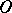with perfect field of residues. Ifis a smooth geometrically non-degenerate singular curve of genus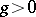over, then its minimal model represents the functor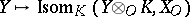from the category of regular-schemes. Ifis an Abelian variety over, then its minimal Néron model is a smooth group scheme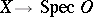, representing the functor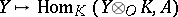from the category of smooth-schemes.

How to Cite This Entry:
Representable functor. Encyclopedia of Mathematics. URL: http://encyclopediaofmath.org/index.php?title=Representable_functor&oldid=11965
This article was adapted from an original article by S.G. TankeevM.Sh. Tsalenko (originator), which appeared in Encyclopedia of Mathematics - ISBN 1402006098. See original article